Next: A note on image Up: Orthogonal moments Previous: Legendre moments

## Complex Zernike moments

The Zernike polynomials were first proposed in 1934 by Zernike . Their moment formulation appears to be one of the most popular, outperforming the alternatives  (in terms of noise resilience, information redundancy and reconstruction capability). The pseudo-Zernike formulation proposed by Bhatia and Wolf  further improved these characteristics. However, here we study the original formulation of these orthogonal invariant moments.

Complex Zernike moments  are constructed using a set of complex polynomials which form a complete orthogonal basis set defined on the unit disc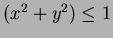. They are expressed as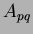Two dimensional Zernike moment:(49)

where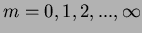and defines the order,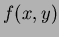is the function being described and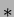denotes the complex conjugate. While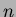is an integer (that can be positive or negative) depicting the angular dependence, or rotation, subject to the conditions:(50)

and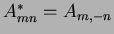is true. The Zernike polynomials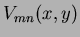Zernike polynomial expressed in polar coordinates are: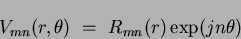(51)

where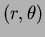are defined over the unit disc,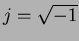and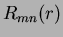is the orthogonal radial polynomial, defined asOrthogonal radial polynomial: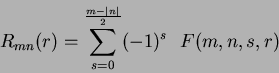(52)

where:(53)

whereand it must be noted that if the conditions in Equation 1.50 are not met, then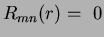. The first six orthogonal radial polynomials are: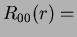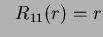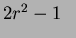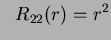(54)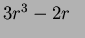Figure 1.6 shows eight such radial responses, where it can been seen that the polynomials become more grouped, as they approach the edge of the unit disc (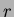approaches unity). (Care must be taken with regard to the accuracy of these polynomial calculations as the factorial operations can quickly produce large integer values, even at relatively low order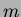). The difference between these orthogonal polynomials and the non-orthogonal monomials can be seen by comparing Figure 1.6 with Figure 1.2.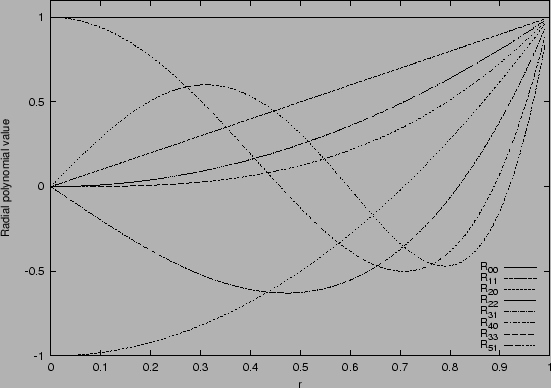So for a discrete image, if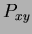is the current pixel then Equation 1.49 becomes: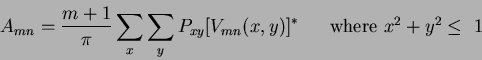(55)

To calculate the Zernike moments, the image (or region of interest) is first mapped to the unit disc using polar coordinates, where the centre of the image is the origin of the unit disc. Those pixels falling outside the unit disc are not used in the calculation. The coordinates are then described by the length of the vector from the origin to the coordinate point,, and the angle from the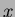axis to the vectorPolar co-ordinate radius,Polar co-ordinate angle, by convention measured from the positiveaxis in a counter clockwise direction. The mapping from Cartesian to polar coordinates is:(56)

where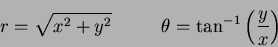(57)

However,in practice is often defined over the interval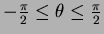, so care must be taken as to which quadrant the Cartesian coordinates appear in. Translation and scale invariance can be achieved by normalising the image using the Cartesian moments prior to calculation of the Zernike moments . Translation invariance is achieved by moving the origin to the image's COM, causing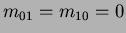. Following this, scale invariance is produced by altering each object so that its area (or pixel count for a binary image) is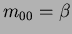, whereis a predetermined value. Both invariance properties (for a binary image) can be achieved using :(58)

andis the new translated and scaled function. The error involved in the discrete implementation can be reduced by interpolation. If the coordinate calculated by Equation 1.58 does not coincide with an actual grid location, the pixel value associated with it is interpolated from the four surrounding pixels. As a result of the normalisation, the Zernike moments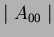and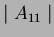are set to known values.is set to zero, due to the translation of the shape to the centre of the coordinate system. This however will be affected by a discrete implementation where the error in the mapping will decrease as the shape (being mapped) size (or pixel-resolution) increases.is dependent on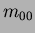, and thus on:(59)

Further, the absolute value of a Zernike moment is rotation invariant as reflected in the mapping of the image to the unit disc. The rotation of the shape around the unit disc is expressed as a phase change, ifis the angle of rotation,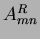is the Zernike moment of the rotated image and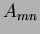is the Zernike moment of the original image then: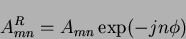(60)

SubsectionsNext: A note on image Up: Orthogonal moments Previous: Legendre moments
Jamie Shutler 2002-08-15